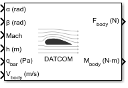Documentation

This is machine translation

Mouseover text to see original. Click the button below to return to the English version of the page.

Digital DATCOM Forces and Moments

Compute aerodynamic forces and moments using Digital DATCOM static and dynamic stability derivatives

Library

AerodynamicsDescription

The Digital DATCOM Forces and Moments block computes the aerodynamic forces and moments about the center of gravity using aerodynamic coefficients from Digital DATCOM.

Algorithms for calculating forces and moments build up the overall aerodynamic forces and moments (F and M) from data contained in the Digital DATCOM structure parameter:

F = Fstatic + Fdyn

M = Mstatic + Mdyn

Fstatic and Mstatic are the static contribution, and Fdyn and Mdyn the dynamic contribution, to the aerodynamic coefficients. If the dynamic characteristics are not contained in the Digital DATCOM structure parameter, their contribution is set to zero.

Static Stability Characteristics

Static stability characteristics include the following.

CoefficientMeaning
CDMatrix of drag coefficients. These coefficients are defined positive for an aft-acting load.
CLMatrix of lift coefficients. These coefficients are defined positive for an up-acting load.
CmMatrix of pitching-moment coefficients. These coefficients are defined positive for a nose-up rotation.
CMatrix of derivatives of side-force coefficients with respect to sideslip angle
CMatrix of derivatives of yawing-moment coefficients with respect to sideslip angle
CMatrix of derivatives of rolling-moment coefficients with respect to sideslip angle

These are the static contributions to the aerodynamic coefficients in stability axes.

CD static = CD

Cy static = Cβ

CL static = CL

Cl static = Cβ

Cm static = CM

Cn static = Cβ

Dynamic Stability Characteristics

Dynamic stability characteristics include the following.

CoefficientMeaning
CLqMatrix of lift force derivatives due to pitch rate
CmqMatrix of pitching-moment derivatives due to pitch rate
CLdα/dtMatrix of lift force derivatives due to rate of angle of attack
Cmdα/dtMatrix of pitching-moment derivatives due to rate of angle of attack
ClpMatrix of rolling-moment derivatives due to roll rate
CYpMatrix of lateral force derivatives due to roll rate
CnpMatrix of yawing-moment derivatives due to roll rate
CnrMatrix of yawing-moment derivatives due to yaw rate
ClrMatrix of rolling-moment derivatives due to yaw rate

These are the dynamic contributions to the aerodynamic coefficients in stability axes.

Parameters

Units

Specifies the input and output units:

Units

Force

Moment

Length

Velocity

Pressure

`Metric (MKS)`

Newton

Newton-
meter

Meters

Meters per second

Pascal

`English (Velocity in ft/s)`

Pound

Foot-pound

Feet

Feet per second

Pound per square inch

`English (Velocity in kts)`

Pound

Foot-pound

Feet

Knots

Pound per square inch

Digital DATCOM structure

Specifies the MATLAB® structure containing the digital DATCOM data. This structure is generated by the Aerospace Toolbox function `datcomimport`. To include dynamic derivatives in the generated output file, call the `datomimport` function with the damp keyword. The Digital DATCOM Forces and Moments block supports only Digital DATCOM, which is the 1976 version of DATCOM.

For more information on creating the digital DATCOM structure, see Importing from USAF Digital DATCOM Files (Aerospace Toolbox). This example shows how to bring United States Air Force (USAF) Digital DATCOM files into the MATLAB environment using the Aerospace Toolbox software.

Force axes

Specifies coordinate system for aerodynamic force: `Body` or `Wind`.

Interpolation method

`None (flat)` or `Linear`

Extrapolation method

`None (clip)` or `Linear`

Process out of range input

Specifies how to handle out-of-range input: ```Linear Extrapolation``` or `Clip to Range`.

Action for out-of-range input

Specifies if out-of-range input invokes a warning, an error, or no action.

Inputs and Outputs

InputDimension TypeDescription

First

ScalarContains the angle of attack.

Second

ScalarContains the sideslip angle, in radians.

Third

ScalarContains the Mach number.

Fourth

ScalarContains the altitude, in selected length units.

Fifth

ScalarContains the dynamic pressure, in selected pressure units.

Sixth

Three-element vectorContains the velocity, in selected velocity units and selected force axes.

Seventh (Optional)

ScalarContains the angle of attack rate, in radians per second. Appears when DAMP Control Card is used in input to Digital DATCOM.

Eight (Optional)

Three-element vectorContains the body angular rates, in radians per second. Appears when DAMP Control Card is used in input to Digital DATCOM.

Ninth (Optional)

ScalarContains the ground height, in select units of length. Appears when GRNDEF Namelist is used in input to Digital DATCOM.

Tenth (Optional)

Contains the control surface deflections, radians. Appears when ASYFLP or SYMFLP and GRNDEF namelists are used in input to Digital DATCOM.
OutputDimension TypeDescription

First

Three-element vectorContains the aerodynamic forces at the center of gravity in selected coordinate system: Body (Fx, Fy, and Fz), or Wind (FD, Fy, and FL).

Second

Three-element vectorContains the aerodynamic moments at the center of gravity in body coordinates (Mx, My, and Mz).

Assumptions and Limitations

The operational limitations of Digital DATCOM apply to the data contained in the Digital DATCOM structure parameter. For more information on Digital DATCOM limitations, see Section 2.4.5 of reference .

The Digital DATCOM structure parameters `alpha`, `mach`, `alt`, `grndht`, and `delta` must be strictly monotonically increasing to be used with the Digital DATCOM Forces and Moments block.

The Digital DATCOM structure coefficients must correspond to the dimensions of the breakpoints (`alpha`, `mach`, `alt`, `grndht`, and `delta`) to be used with the Digital DATCOM Forces and Moments block.

References

 The USAF Stability and Control Digital Datcom, AFFDL-TR-79-3032, 1979.

 Etkin, B., and L. D. Reid, Dynamics of Flight Stability and Control, John Wiley & Sons, New York, 1996.

 Roskam, J., “Airplane Design Part VI: Preliminary Calculation of Aerodynamic, Thrust and Power Characteristics,” Roskam Aviation and Engineering Corporation, Ottawa, Kansas, 1987.

 Stevens, B. L., and F. L. Lewis, Aircraft Control and Simulation, John Wiley & Sons, New York, 1992.

Example

See Importing from USAF Digital DATCOM Files (Aerospace Toolbox) for an example of how to create and format DATCOM data for this block.

See `asbSkyHogg` for an example of this block.

Introduced in R2006b

Aerospace Blockset DocumentationGet trial now